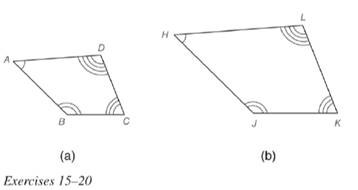Chapter 5.2, Problem 18E### Elementary Geometry for College St...

6th Edition
Daniel C. Alexander + 1 other
ISBN: 9781285195698

#### Solutions

Chapter
Section### Elementary Geometry for College St...

6th Edition
Daniel C. Alexander + 1 other
ISBN: 9781285195698
Textbook Problem
46 views

# Quadrilateral A B C D ∼ quadrilateral H J K L . If m ∠ D = 90 ∘ , A D = 8 , D C = 6 , and H L = 12 , find the length of diagonal H K ¯ (not shown).To determine

To calculate:

The value of diagonal HK¯.

Explanation

Given:

Concept Used:

The sum of measure of angle in a quadrilateral is 360.

Similarity between Two polygons:

Two polygons are similar if and only if when all pairs of corresponding angles are congruent and all pairs of corresponding sides are proportional.

Pythagoras Theorem:

It states that sum of the length of the two sides is equal to the square of the length of the hypotenuse (longest sides).

(Hypotenuse)2=(Base)2+(Perpendicular)2

Calculation:

According to the definition of similar polygon, AH,BJ,DL,and CK

Substitute the value of LK,DC,HL and AD in an above equation, we get

LK6=128LK=1268=9

It is given that mD=90

### Still sussing out bartleby?

Check out a sample textbook solution.

See a sample solution

#### The Solution to Your Study Problems

Bartleby provides explanations to thousands of textbook problems written by our experts, many with advanced degrees!

Get Started

#### x2 2x 5 = 0

Applied Calculus for the Managerial, Life, and Social Sciences: A Brief Approach

#### In problems 1-16, solve each equation. 7. Solve

Mathematical Applications for the Management, Life, and Social Sciences

#### The slope of the tangent line to y = x3 at x = 2 is: 18 12 6 0

Study Guide for Stewart's Single Variable Calculus: Early Transcendentals, 8th